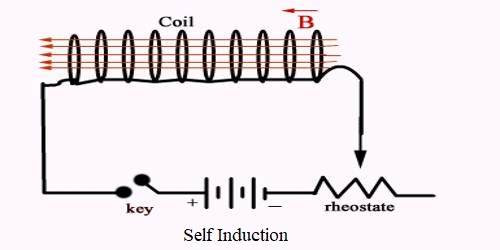# Self Induction

Self Induction

When current flows through a closed coil, then that current creates a magnetic field and the coil becomes associated with that field. So, if there is a change of current in the coil, associated magnetic field lines also change, and induced electromotive force is produced in the coil which opposes the very change of current. It is called self-induction. It is the induction of an electromotive force in a circuit when the current in that circuit is varied.In figure an electric circuit is shown by connecting a coil L, an electric cell E, and a tapping key K. If the tapping key is pressed, current starts flowing in the circuit and reaches to a steady value. To reach this steady value time ‘0’ to ‘t’ is needed. During this time ‘t’ due to the increase of current in the coil, there is an increase of magnetic field lines or flux. Consequently, according to Faraday’s law of electromagnetic induction, current flows in the circuit which opposes the principal current. When the current reaches a steady value, the flux in the coil does not change; hence no induced current exists in the circuit. Again, when the tapping key is released i.e., the circuit is disconnected; it takes some time for the current to come down to zero value. During this time induced current is produced and as the direction of this induced current is in the same direction of the principal current, so it opposes file decrease of the principal current. The above phenomenon is called self induction.

That means the electromagnetic induction which is produced in a single closed coil due to the change of magnetic flux which results for the non-uniform current or due to the motion of the closed coil in the magnetic field is called self induction.